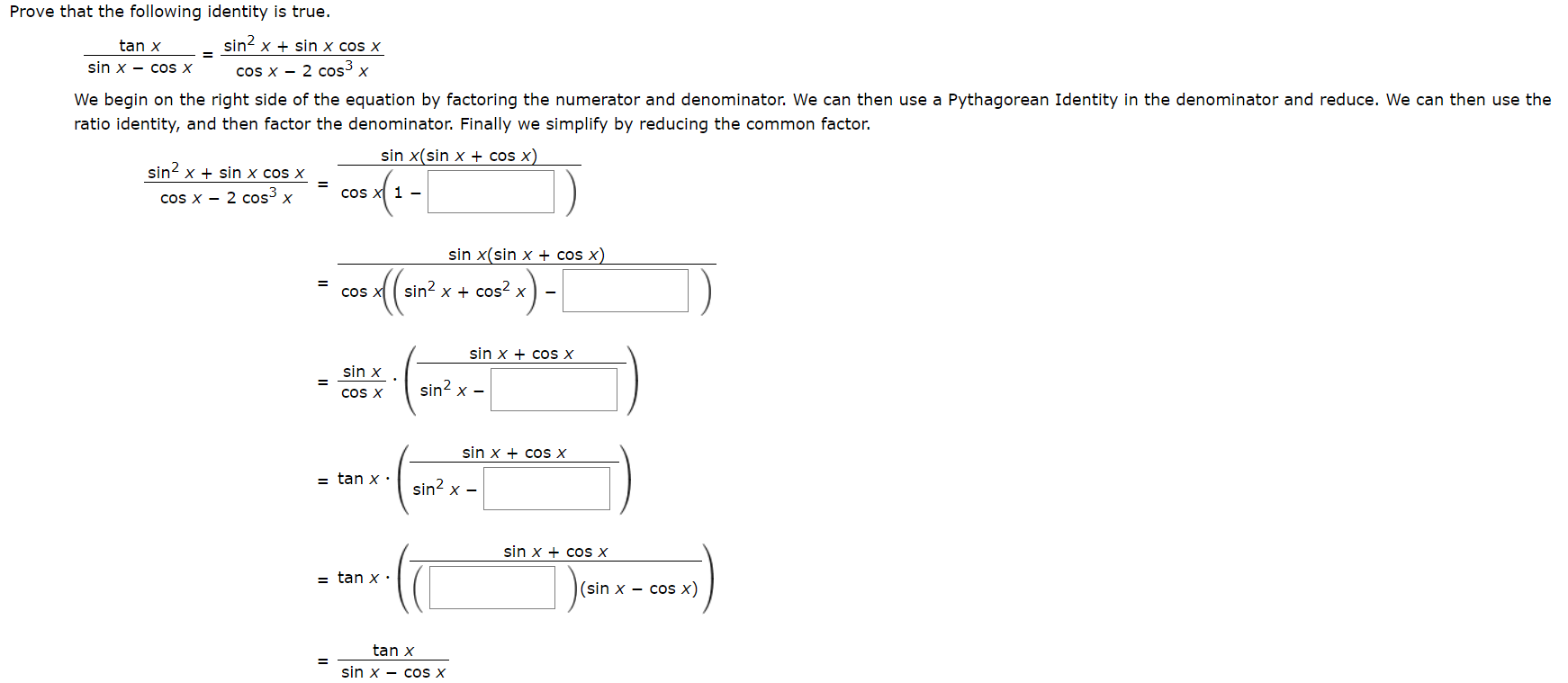# Prove that the following identity is true.sin2tan xx sin x cos x=sin x - cos xcos x 2 cos xWe begin on the right side of the equation by factoring the numerator and denominator. We can then use a Pythagorean Identity in the denominator and reduce. We can then use theratio identity, and then factor the denominator. Finally we simplify by reducing the common factor.sin x(sin x + cos x)sin2 xsin x cos xCos X 1 -cos X 2 cos3 xcos x)sin x(sin x=sin2 xcos2 xCOS Xsin xcos xsin xsin2 x -COS Xsin x cos x= tan xsin2 xsin x cos x= tan x.(sin x - cos x)tan xsin x - cos x

Question
2 views

photo attachedhelp_outlineImage TranscriptioncloseProve that the following identity is true. sin2 tan x x sin x cos x = sin x - cos x cos x 2 cos x We begin on the right side of the equation by factoring the numerator and denominator. We can then use a Pythagorean Identity in the denominator and reduce. We can then use the ratio identity, and then factor the denominator. Finally we simplify by reducing the common factor. sin x(sin x + cos x) sin2 xsin x cos x Cos X 1 - cos X 2 cos3 x cos x) sin x(sin x = sin2 xcos2 x COS X sin x cos x sin x sin2 x - COS X sin x cos x = tan x sin2 x sin x cos x = tan x. (sin x - cos x) tan x sin x - cos x fullscreen
check_circle

Step 1

Given informa...

### Want to see the full answer?

See Solution

#### Want to see this answer and more?

Solutions are written by subject experts who are available 24/7. Questions are typically answered within 1 hour.*

See Solution
*Response times may vary by subject and question.
Tagged in
MathTrigonometry

### Trigonometric Ratios